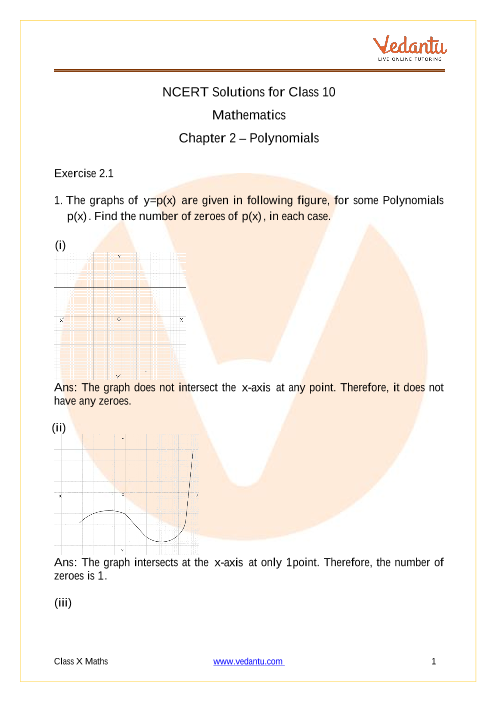# NCERT Solutions for Class 10 Maths Chapter 2 Polynomials

## NCERT Solutions for Class 10 Maths Chapter 2 Polynomials - Free PDF

NCERT Solutions Class 10 Maths Chapter 2 Polynomial prepared by Vedantu’s proficient subject experts aims to deliver an optimum and in-depth analysis of the chapter. It is in accordance with the exam specifications and adheres to the latest syllabus formulated by CBSE. Considering the doubts and problems faced by the students and teachers, it incorporates the concise, brief and self-explanatory solutions along with key facts and formulae to aid your conceptual understanding and provide you sufficient practise to achieve sure success. You will get the download option for NCERT Class 10 Maths Solution for Chapter 2 Polynomials in PDF format and store it in any of your preferred devices. NCERT Solutions for all subjects and classes are available on Vedantu. Science students who are looking for NCERT Solutions for Class 10 Science will also find the solutions curated by our Master Teachers really helpful.Q1. What is the difference between remainder theorem and factor theorem?

Remainder theorem states that if a polynomial p(x) is divided by (x - a), then the remainder is obtained by evaluating the expression  p(a).

While, Factor theorem states that (x - a) will be a factor of polynomial p(x) only if the remainder obtained by evaluating the expression p(a) equals zero i.e; p(a) = 0.

For example: let p(x) = x² - 6x + 9, then find the remainder when it is divided by (x - 1).

So, according to the remainder theorem, remainder is obtained by evaluating the expression p(1)

p(1) = 1² - 6(1) + 9

= 1 - 6 + 9 = 4.

Therefore, the remainder is 4 when  p(x) = x² - 6x + 9 is divided by (x - 1).

Now, check whether (x - 3) is the factor of p(x) = x² - 6x + 9.

So, according to factor theorem  (x - 3) is the factor of p(x) = x² - 6x + 9 only if p(3) = 0.

p(3) = 3² - 6(3) + 9

= 9 - 18 + 9 = 0.

Therefore, (x - 3) is the factor of p(x) = x² - 6x + 9.

Q2:  What is the geometrical meaning of the zeroes of a Polynomial?

1. For a linear polynomial ax + b, a ≠ 0, the graph of y = ax + b is a straight line which intersects the x-axis at exactly one point at (-b/a , 0). Therefore, the geometrical meaning of the zeroes of linear polynomial ax + b, a ≠ 0 is the  x-coordinate of the point where the graph of y = ax + b intersects the x-axis.

2. For a quadratic polynomial ax² + bx + c, a ≠ 0, the graph of the equation y = ax² + bx + c is parabola, which is ‘U’ shaped either open upwards or open downwards depending on whether a > 0 or a < 0. Therefore, the geometrical meaning of a quadratic polynomial is that it can have either two distinct zeroes or two equal zeroes (i.e., one zero), or no zero. This means that a polynomial of degree 2 has at most two zeroes.

In general, for any given polynomial p(x) of degree n, the graph of y = p(x) intersects the x-axis at atmost n points. Therefore, the geometrical meaning for a polynomial p(x) of degree n is that it has at most n zeroes.

Q3. What are the relationships between zeroes and coefficients of (a) Quadratic polynomial (b) Cubic polynomial?

1. For quadratic polynomial ax² + bx + c, a ≠ 0, if α and β are its zeroes, then relationship between the zeroes and coefficients of quadratic polynomial is:

1. Sum of zeroes = α + β = -b/a = -(coefficient of x) / coefficient of x²

2. Product of zeroes = αβ = c/a = constant term / coefficient of x².

1. For cubic polynomial ax³ + bx² + cx + d, a ≠ 0, if α, β and γ are its zeroes, then relationship between the zeroes and coefficients of cubic polynomial is:

1. Sum of zeroes = α + β + γ = -b/a = -(coefficient of x²) / coefficient of x³.

2. Sum of product of zeroes taken two at a time = αβ + βγ + γα = c/a = coefficient of x / coefficient of x³.

3. Product of zeroes =  α β γ = d/a = constant term / coefficient of x³.Do you wish to have an edge over others?